# Seat

How much m² of fabric do we need to sew a 50cm-shaped cube-shaped seat if 10% of the material we add to the folds?

X =  1.65 m2

### Step-by-step explanation: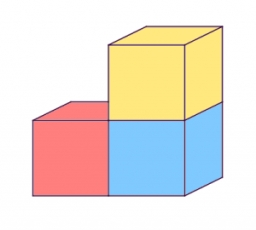Did you find an error or inaccuracy? Feel free to write us. Thank you!Tips to related online calculators

#### You need to know the following knowledge to solve this word math problem:

We encourage you to watch this tutorial video on this math problem:

## Related math problems and questions:

• Metal sheet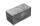How much m ^ 2 of sheet metal is needed to beat a box in the shape of a cube with an edge length of 50 cm, if we add 20% on the folds of the lid and walls?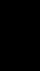The cone-shaped lampshade has a diameter of 30 cm and a height of 10 cm. How many cm2 of material will we need when we 10% is waste?Marie wants to make a cone-shaped witch's hat for a masquerade ball. How much material will it need if it counts on an annular rim with diameters of 28cm and 44cm? Hat side length is 30cm. Add 5% of the material to the bust. Round to cm2.
• Sphere vs cubeHow many % of the surface of a sphere of radius 12 cm is the surface of a cube inscribed in this sphere?
• Cylinder in cube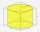Into a paper box in the shape of a cube with an edge of 10 cm is placed a can in the shape of a cylinder with a height of 10 cm and touching all the walls of the cube. What % of the volume of the cube does the can take up?
• Surface area 2Calculate how many % reduce the surface area of the cube is we reduced length of each edge by 10%.
• PaintingThe room is long 50 meters and wide 60dm and 300 cm high. Calculate how much it will cost painting if area of windows and doors is 15% of the total area. One square meter cost 50cents.
• Cone roofHow many m2 of roofing is needed to cover a cone-shaped roof with a diameter of 10 m and a height of 4 m? Add an extra 4% to the overlays.
• Iglu - cone tentThe cone-shaped tent is 3 m high, the diameter of its base is 3.2 m. a) The tent is made of two layers of material. How many m2 of fabric is needed for production (including flooring), if 20% needs to be added to the minimum amount due to cutting waste? b
• GutterHow much metal is needed for production 60 pieces of gutter pipes with the diameter 17 cm and length of 6 m? The plate bends add 6% of the material.
• Cuboid boxHow many m2 paper is needed for the sticking cuboid box of dimensions 50 cm, 40 cm and 30 cm? To the folds add one-tenth the area.
• Minimum surfaceFind the length, breadth, and height of the cuboid-shaped box with a minimum surface area, into which 50 cuboid-shaped blocks, each with length, breadth, and height equal to 4 cm, 3 cm, and 2 cm respectively, can be packed.
• Metal box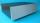How much metal we need for the production of box with dimensions 5 dm, 30 cm and a height of 1 m? Add 12% of the waste and folds.
• Content area and percentsDetermine what percentage is smaller cube surface, when the surface area of the wall decreases by 25%.
• The body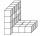The body on the figure consists of cubes with an edge length 10 cm. What surface has this body?
• Cube surface areaThe surface of the cube was originally 216 centimeters square. The surface of the cube has shrunk from 216 to 54 centimeters sq. Calculate how much percent the edge of the cube has decreased.
• BoxCardboard box-shaped quadrangular prism with a rhombic base. Rhombus has a side 5 cm, and one diagonal 8 cm long, and the box's height is 12 cm. The box will open at the top. How many cm2 of cardboard do we need to cover overlap and joints that are 5% of